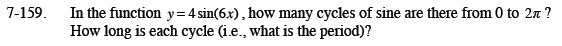Home > CCA2 > Chapter 7 > Lesson 7.2.4 > Problem7-159

7-159.

In the function y = 4sin(6x), how many cycles of sine are there from 0 to 2π? How long is each cycle (i.e., what is the period)? Homework Help ✎The number of cycles is b in the equation. The length of each cycle is p (the period) where: